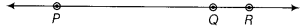# If P,Q,R are three points on a line and Q is between P and R, prove that PR - QR = PQ

If P,Q,R are three points on a line and Q is between P and R, then prove that PR - QR = PQ.

In the given figure, PQ coincides with PR-QR.So, according to axiom, ‘things’ which coincide with one another, are equal to one another’.
∴ PR-QR = PQ